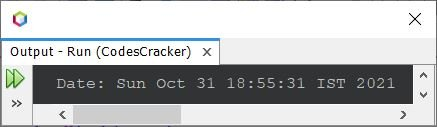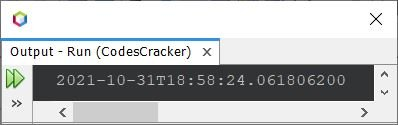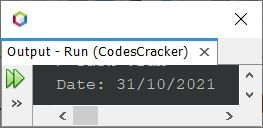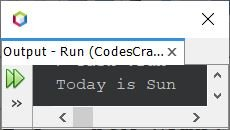# Java Program to Print Date and Time

This article covers multiple programs in Java that prints the current date and time. Here are the list of programs covered in this article:

• Get and print the complete current date in default format
• Get and print the current date, in specified format
• Get and print the current time, in specified format
• Print today's day
• Print time, day, and date in specified format

## Get and Print Current Date in Java

The question is, write a Java program to print the current date. The program given below is its answer. This program prints the complete current date in default format.

```import java.util.Date;

public class CodesCracker
{
public static void main(String[] args)
{
Date d = new Date();
System.out.println("Date: " +d);
}
}```

The snapshot given below shows the sample output produced by above program, on printing the current date:Here is another way to create a program in Java that does the same job as of previous program using now().

```import java.time.LocalDateTime;

public class CodesCracker
{
public static void main(String[] args)
{
System.out.println(LocalDateTime.now());
}
}```

#### Sample Output of Previous Program## Print Only Date in Specified Format in Java

This program only prints the current date, in of course, a specified or given format. I've used the format / to separate day, month, and year. You can use any other character to format the date such as dash (-), comma (,) etc.

```import java.util.*;
import java.text.*;

public class CodesCracker
{
public static void main(String[] args)
{
Date d = new Date();
SimpleDateFormat a = new SimpleDateFormat("dd/MM/yyyy");
System.out.println("Date: " +a.format(d));
}
}```

#### Sample Output of Previous Program## Print Only Time in Specified Format in Java

This program prints the current time only, in again specified format.

```import java.util.*;
import java.text.*;

public class CodesCracker
{
public static void main(String[] args)
{
Date d = new Date();
SimpleDateFormat a = new SimpleDateFormat("hh:mm:ss a");
System.out.println("The Current Time is " +a.format(d));
}
}```

#### Sample Output of Previous Program## Print Today's Day in Java

This program prints today's day in Java.

```import java.util.*;
import java.text.*;

public class CodesCracker
{
public static void main(String[] args)
{
Date d = new Date();
SimpleDateFormat a = new SimpleDateFormat("E");
System.out.println("Today is " +a.format(d));
}
}```

#### Sample Output of Previous Program## Print Current Time, Day, and Date in Java

This is the last program of this article, created after combining the previous three programs, to find and print the current time, day, and date.

```import java.util.*;
import java.text.*;

public class CodesCracker
{
public static void main(String[] args)
{
Date d = new Date();

SimpleDateFormat a = new SimpleDateFormat("E");
SimpleDateFormat b = new SimpleDateFormat("hh:mm a");
SimpleDateFormat c = new SimpleDateFormat("dd-MM-yyyy");

System.out.println("Today is \"" +a.format(d)+ "\"");
System.out.println("The Current Time is \"" +b.format(d)+ "\"");
System.out.println("The Current Date is \"" +c.format(d)+ "\"");
}
}```

#### Sample Output of Previous Program#### Same Program in Other Languages

Java Online Test

« Previous Program Next Program »